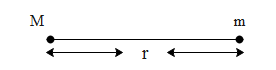QuestionAnswers

# The force of attraction between two bodies(A,B) depends upona) mass of Ab) mass of Bc) distance between themd) All of theseVerified
131.4k+ views
Hint: To draw a conclusion first we need to know how the force of attraction is defined and on what does it depend upon. We will use Newton's Law of gravitation to define the force of attraction between any two bodies. After we obtain a result from the law of gravitation, we will compare the result and see on what quantities mentioned in the options does it depend on.Hence mathematically this law can be written as, $\text{F}= -\dfrac{\text{GMm}}{{{\text{r}}^{\text{2}}}}\text{N}$.
The value of G in space is $\text{6}\text{.67 }\!\!\times\!\!\text{ 1}{{\text{0}}^{\text{-11}}}\text{ N}{{\text{m}}^{\text{2}}}\text{ k}{{\text{g}}^{\text{-2}}}$. From this itself we can conclude that the gravitational force is very weak in nature and even if the force exists between any two bodies having mass there will be no net motion of the body. This force will only result in motion between two bodies if the mass of either one or both the bodies is of the order greater than $\text{1}{{\text{0}}^{\text{-11}}}$.